# Class 11 Maths NCERT Solutions for Chapter 16 Probability Exercise 16.3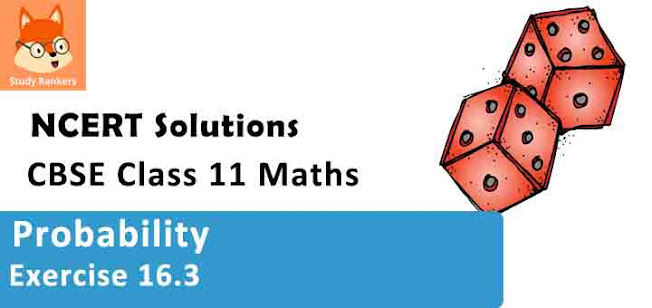### Statistics Exercise 16.3 Solutions

1.  Which of the following can not be valid assignment of probabilities for outcomes of sample space S = {ω1, ω2,ω3,ω4,ω5,ω6,ω7}

 Assignment ω1 ω2 ω3 ω4 ω5 ω6 ω7 (a) 0.1 0.01 0.05 0.03 0.01 0.2 0.6 (b) 1/7 1/7 1/7 1/7 1/7 1/7 1/7 (c) 0.1 0.2 0.3 0.4 0.5 0.6 0.7 (d) –0.1 0.2 0.3 0.4 –0.2 0.1 0.3 (e) 1/14 2/14 3/14 4/14 5/14 6/14 15/14

Solution
(a)
 ω1 ω2 ω3 ω4 ω5 ω6 ω7 0.1 0.01 0.05 0.03 0.01 0.2 0.6
Here, each of the numbers pi) is positive and less than 1.
Sum of probabilities
= p(ω1 ) + p(ω2 ) + p(ω3) + p(ω4) + p(ω5) + p(ω6) + p(ω7)
= 0.1 + 0.01 + 0.05 + 0.03 + 0.01 + 0.2 + 0.6
= 1
Thus, the assignment is valid.

(b)
 ω1 ω2 ω3 ω4 ω5 ω6 ω7 1/7 1/7 1/7 1/7 1/7 1/7 1/7
Here, each of the numbers pi) is positive and less than 1.
Sum of probabilities
= p(ω1 ) + p(ω2 ) + p(ω3) + p(ω4) + p(ω5) + p(ω6) + p(ω7)
= 1/7 + 1/7 + 1/7 + 1/7 + 1/7 + 1/7 +1/7 = 7 × 1/7 = 1
Thus, the assignment is valid.

(c)
 ω1 ω2 ω3 ω4 ω5 ω6 ω7 0.1 0.2 0.3 0.4 0.5 0.6 0.7
Here, each of the numbers pi) is positive and less than 1.
Sum of probabilities
= p(ω1 ) + p(ω2 ) + p(ω3) + p(ω4) + p(ω5) + p(ω6) + p(ω7
= 0.1 + 0.2 + 0.3 + 0.4 + 0.5 + 0.6 + 0.7
= 2.8 ≠ 1
Thus, the assignment is not valid.

(d)
 ω1 ω2 ω3 ω4 ω5 ω6 ω7 –0.1 0.2 0.3 0.4 –0.2 0.1 0.3
Here, p1) and p5) are negative.
Hence, the assignment is not valid.

(e)
 ω1 ω2 ω3 ω4 ω5 ω6 ω7 1/14 2/14 3/14 4/14 5/14 6/14 15/14
Here, p(ω7 ) = 15/14 > 1
Hence, the assignment is not valid.

2. A coin is tossed twice, what is the probability that at least one tail occurs?
Solution

When a coin is tossed twice, the sample space is given by
S = {HH, HT, TH, TT}
Let A be the event of the occurrence of at least one tail.
Accordingly, A = {HT, TH, TT}
∴ P(A) = (Number of outcomes favourable to A)/(Total number of possible outcomes)
= n(A)/n(S)
= 3/4

3. A die is thrown, find the probability of following events:
(i) A prime number will appear,
(ii) A number greater than or equal to 3 will appear,
(iii) A number less than or equal to one will appear,
(iv) A number more than 6 will appear,
(v) A number less than 6 will appear.

Solution

The sample space of the given experiment is given by
S = {1, 2, 3, 4, 5, 6}

(i) Let A be the event of the occurrence of a prime number.
Accordingly, A = {2, 3, 5}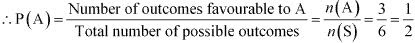(ii) Let B be the event of the occurrence of a number greater than or equal to 3.
Accordingly, B = {3, 4, 5, 6}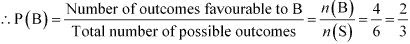(iii) Let C be the event of the occurrence of a number less than or equal to one.
Accordingly, C = {1}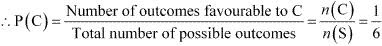(iv) Let D be the event of the occurrence of a number greater than  6.
Accordingly, D = Φ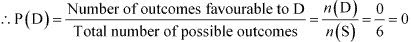(v) Let E be the event of the occurrence of  a number less than 6.
Accordingly, E = {1, 2, 3, 4, 5}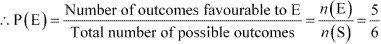4. A card is selected from a pack of 52 cards.
(a) How many points are there in the sample space?
(b) Calculate the probability that the card is an ace of spades.
(c) Calculate the probability that the card is (i) an ace (ii) black card.

Solution

(a) When a card is selected from a pack of 52 cards, the number of possible outcomes is 52 i.e., the sample space contains 52 elements.
Therefore, there are 52 points in the sample space.

(b) Let A be the event in which the card drawn is an ace of spades.
Accordingly, n(A) = 1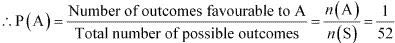(c) (i) Let E be the event in which the card drawn is an ace.
Since there are 4 aces in a pack of 52  cards, n(E) = 4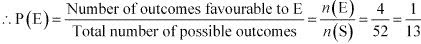(ii) Let F be the event in which the card drawn is black.
Since there are  26 black cards in a pack of 52 cards, n(F) = 26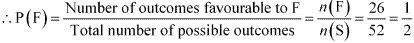5. A fair coin with 1 marked on one face and 6 on the other and a fair die are both tossed. Find the probability that the sum of numbers that turn up is (i) 3 (ii) 12

Solution

Since the fair coin has 1 marked on one face and 6 on the other, and the die has six faces that are numbered 1, 2, 3, 4, 5, and 6, the sample space is given by
S = {(1, 1), (1, 2), (1, 3), (1, 4), (1, 5), (1, 6), (6, 1), (6, 2), (6, 3), (6, 4), (6, 5), (6, 6)}
Accordingly, n(S) = 12

(i) Let A be the event in which the sum of numbers that turn up is 3.
Accordingly, A = {(1, 2)}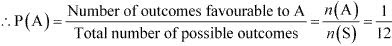(ii) Let B be the event in which the sum of numbers that turn up is 12.
Accordingly, B = {(6, 6)}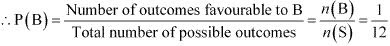6. There are four men and six women on the city council. If one council member is selected for a committee at random, how likely is it that it is a woman?

Solution

There are four men and six women on the city council.
As one council member is to be selected for a committee at random, the sample space contains 10 (4 + 6) elements.
Let A be the event in which the selected council member is a woman.
Accordingly, n(A) = 6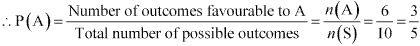7. A fair coin is tossed four times, and a person win Re 1 for each head and lose Rs 1.50 for each tail that turns up. From the sample space calculate how many different amounts of money you can have after four tosses and the probability of having each of these amounts.

Solution

Since the coin is tossed four times, there can be a maximum of 4 heads or tails.
When 4 heads turns up, Re 1 + Re 1 + Re 1+ Re 1 = Rs 4 is the gain.
When 3 heads and 1 tail turn up, Re 1 + Re 1 + Re 1 – Rs 1.50 = Rs 3 – Rs 1.50 = Rs 1.50 is the gain.
When 2 heads and 2 tails turns up, Re 1 + Re 1 – Rs 1.50 – Rs 1.50 = – Re 1, i.e., Re 1 is the loss.
When 1 head and 3 tails turn up, Re 1 – Rs 1.50 – Rs 1.50 – Rs 1.50 = – Rs 3.50, i.e., Rs 3.50 is the loss.
When 4 tails turn up, – Rs 1.50 – Rs 1.50 – Rs 1.50 – Rs 1.50 = – Rs 6.00, i.e., Rs 6.00 is the loss.
There are 24 = 16 elements in the sample space S, which is given by:
S = {HHHH, HHHT, HHTH, HTHH, THHH, HHTT, HTTH, TTHH, HTHT, THTH, THHT, HTTT, THTT, TTHT, TTTH, TTTT}
∴ n(S) = 16
The person wins Rs 4.00 when 4 heads turn up, i.e., when the event {HHHH} occurs.
∴ Probability (of winning Rs 4.00) =1/16
The person wins Rs 1.50 when 3 heads and one tail turn up, i.e., when the event {HHHT, HHTH, HTHH, THHH} occurs.
∴ Probability (of winning Rs 1.50) = 4/16 = 1/4
The person loses Re 1.00 when 2 heads and 2 tails turn up, i.e., when the event {HHTT, HTTH, TTHH, HTHT, THTH, THHT} occurs.
∴ Probability (of losing Re 1.00) = 6/16 = 3/8
The person loses Rs 3.50 when 1 head and 3 tails turn up, i.e., when the event {HTTT, THTT, TTHT, TTTH} occurs.
Probability (of losing Rs 3.50) = 4/16 = 1/4
The person loses Rs 6.00 when 4 tails turn up, i.e., when the event {TTTT} occurs.
Probability (of losing Rs 6.00) =1/16

8. Three coins are tossed once. Find the probability of getting
(vi) 3 tails
(vii) exactly two tails
(viii) no tail
(ix) at most two tails.

Solution

When three coins are tossed once, the sample space is given by
S = {HHH, HHT, HTH, THH, HTT, THT, TTH, TTT}
∴ Accordingly, n(S) = 8
It is known that the probability of an event A is given by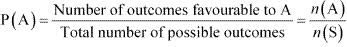(i) Let B be the event of the occurrence of 3 heads. Accordingly, B = {HHH}
∴ P(B) = n(B)/n(S) = 1/8

(ii) Let C be the event of the occurrence of 2 heads. Accordingly, C = {HHT, HTH, THH}
∴ P(C) = n(C)/n(S) = 3/8

(iii) Let D be the event of the occurrence of at least 2 heads.
Accordingly, D = {HHH, HHT, HTH, THH}
∴ P(D) =n(D)/n(S) =4/8 = 1/2

(iv) Let E be the event of the occurrence of at most 2 heads.
Accordingly, E = {HHT, HTH, THH, HTT, THT, TTH, TTT}
∴ P(E) = n(E)/n(S) = 7/8

(v) Let F be the event of the occurrence of no head.
Accordingly, F = {TTT}
∴P(F) =n(F)/n(S) = 1/8

(vi) Let G be the event of the occurrence of 3 tails.
Accordingly, G = {TTT}
∴P(G) = n(G)/n(S) = 1/8

(vii) Let H be the event of the occurrence of exactly 2 tails.
Accordingly, H = {HTT, THT, TTH}
∴P(H) = n(H)/n(S) = 3/8

(viii) Let I be the event of the occurrence of no tail.
Accordingly, I = {HHH}
∴P(I) = n(I)/n(S) = 1/8

(ix) Let J be the event of the occurrence of at most 2 tails.
Accordingly, I = {HHH, HHT, HTH, THH, HTT, THT, TTH}
∴P(J) =n(J)/n(S) = 7/8

9. If 2/11 is the probability of an event, what is the probability of the event ‘not A’.

Solution

It is given that P(A) = 2/11.
Accordingly, P(not A) = 1 - P(A) = 1 - 2/11 = 9/11

10. A letter is chosen at random from the word ‘ASSASSINATION’. Find the probability that letter is (i) a vowel (ii) an consonant

Solution

There are 13 letters in the word ASSASSINATION.
∴Hence, n(S) = 13

(i) There are 6 vowels in the given word.
∴Probability (vowel) = 6/13

(ii) There are 7 consonants in the given word.
∴Probability (consonant) =7/13

11. In a lottery, person choses six different natural numbers at random from 1 to 20, and if these six numbers match with the six numbers already fixed by the lottery committee, he wins the prize. What is the probability of winning the prize in the game? [Hint:order of the numbers is not important.]

Solution

Total number of ways in which one can choose six different numbers from 1 to 20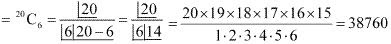Hence, there are 38760 combinations of 6 numbers.
Out of these combinations, one combination is already fixed by the lottery committee.
∴ Required probability of winning the prize in the game = 1/38760

12. Check whether the following probabilities P(A) and P(B) are consistently defined
(i) P(A) = 0.5, P(B) = 0.7, P(A ∩ B) = 0.6
(ii) P(A) = 0.5, P(B) = 0.4, P(A ∪ B) = 0.8

Solution

(i) P(A) = 0.5, P(B) = 0.7, P(A ∩ B) = 0.6
It is known that if E and F are two events such that E ⊂ F, then P(E) ≤ P(F).
However, here, P(A ∩ B) > P(A).
Hence, P(A) and P(B) are not consistently defined.

(ii) P(A) = 0.5, P(B) = 0.4, P(A ∪ B) = 0.8
It is known that if E and F are two events such that E ⊂ F, then P(E) ≤ P(F).
Here, it is seen that P(A ∪ B) > P(A) and P(A ∪ B) > P(B).
Hence, P(A) and P(B) are consistently defined.

13. Fill in the blanks in following table:

 P(A) P(B) P(A ∩ B) P(A ∪ B) (i) 1/3 1/5 1/15 … (ii) 0.35 … 0.25 0.6 (iii) 0.5 0.35 … 0.7

Solution

(i) Here, P(A) = 1/3, P(B) = 1/5, P(A ∩ B) = 1/15
We know that P(A U B) = P(A) + P(B) - P(A ∩ B)
∴ P(A U B) = 1/3 + 1/5 - 1/15 = (5 + 3 - 1)/15 = 7/15

(ii) Here, P(A) = 0.35, P(A ∩ B) = 0.25, P(A ∪ B) = 0.6
We know that P(A ∪ B) = P(A) + P(B) – P(A ∩ B)
∴ 0.6 = 0.35 + P(B) – 0.25
⇒ P(B) = 0.6 – 0.35 + 0.25
⇒ P(B) = 0.5

(iii) Here, P(A) = 0.5, P(B) = 0.35, P(A ∪ B) = 0.7
We know that P(A ∪ B) = P(A) + P(B) – P(A ∩ B)
∴ 0.7 = 0.5 + 0.35 – P(A ∩ B)
⇒ P(A ∩ B) = 0.5 + 0.35 – 0.7
⇒ P(A ∩ B) = 0.15

14. Given P(A) = 3/5 and P(B) = 1/5 . Find P(A or B), if A and B are mutually exclusive events.
Solution
Here, P(A) = 3/5, P(B) = 1/5
For mutually exclusive events A and B,
P(A or B) = P(A) + P(B)
∴ P(A or B) = 3/5 + 1/5 = 4/5

15. If E and F are events such that P(E) =1/4 , P(F) =1/2 and P(E and F) =1/8, find:
(i) P(E or F),
(ii) P(not E and not F).
Solution

Here, P(E) =1/4, P(F) =1/2, and P(E and F) =1/8

(i) We know that P(E or F) = P(E) + P(F) – P(E and F)
∴P(E or F) = 1/4 + 1/2 – 1/8 = (2 + 4 – 1)/8 = 5/8

(ii) From (i), P(E or F) = P (E ∪ F) = 5/8
We have (E ∪ F)' = (E' ∩ F')  [By De Morgan's law]
∴ P(E' ∩ F') = P(E ∪ F)'
Now, P(E ∪ F)' = 1 - P(E ∪ F) = 1 - 5/8 = 3/8
∴ P(E' ∩ F') = 3/8
Thus, P(not E and not F) = 3/8

16. Events E and F are such that P(not E or not F) = 0.25, State whether E and F are mutually exclusive.

Solution

It is given that P(not E or not F) = 0.25
i.e., P(E' ∪ F') = 0.25
⇒ P(E ∩ F)' = 0.25  [E' ∪ F' = (E ∩ F)']
Now, P(E ∩ F) = 1 - P(E ∩ F)'
⇒ P(E ∩ F) =  1 - 0.25
⇒ P(E ∩ F) = 0.75 ≠ 0
⇒ E ∩ F ≠ ∅
Thus, E and F are not mutually exclusive.

17. A and B are events such that P(A) = 0.42, P(B) = 0.48 and P(A and B) = 0.16. Determine
(i) P(not A),
(ii) P (not B) and
(iii) P(A or B).

Solution

It is given that P(A) = 0.42, P(B) = 0.48, P(A and B) = 0.16
(i) P(not A) = 1 – P(A) = 1 – 0.42 = 0.58
(ii) P(not B) = 1 – P(B) = 1 – 0.48 = 0.52
(iii) We know that P(A or B) = P(A) + P(B) – P(A and B)
∴ P(A or B) = 0.42 + 0.48 – 0.16 = 0.74

18. In Class XI of a school 40% of the students study Mathematics and 30% study Biology. 10% of the class study both Mathematics and Biology. If a student is selected at random from the class, find the probability that he will be studying Mathematics or Biology.

Solution

Let A be the event in which the selected student studies Mathematics and B be the event in which the selected student studies Biology.
Accordingly, P(A) = 40% = 40/100 = 2/5
P(B) = 30% = 30/100 = 3/10
P(A and B) = 10% = 10/100 = 1/10
We know that P(A or B) = P(A) + P(B) – P(A and B)
∴ P(A or B) = 2/5 + 3/10 - 1/10 = 6/10 = 0.6
Thus, the probability that the selected student will be studying Mathematics or Biology is 0.6.

19. In an entrance test that is graded on the basis of two examinations, the probability of a randomly chosen student passing the first examination is 0.8 and the probability of passing the second examination is 0.7. The probability of passing at least one of them is 0.95. What is the probability of passing both?

Solution

Let A and B be the events of passing first and second examinations respectively.
Accordingly, P(A) = 0.8, P(B) = 0.7 and P(A or B) = 0.95
We know that P(A or B) = P(A) + P(B) – P(A and B)
∴0.95 = 0.8 + 0.7 – P(A and B)
⇒ P(A and B) = 0.8 + 0.7 – 0.95 = 0.55
Thus, the probability of passing both the examinations is 0.55.

20. The probability that a student will pass the final examination in both English and Hindi is 0.5 and the probability of passing neither is 0.1. If the probability of passing the English examination is 0.75, what is the probability of passing the Hindi examination?

Solution

Let A and B be the events of passing English and Hindi examinations respectively.
Accordingly, P(A and B) = 0.5 , P(not A and not B) = 0.1 , i.e., P(A' ∩ B') = 0.1
P(A) = 0.75
Now, (A ∪ B)' = ( A' ∩ B') [De Morgan's law]
∴ P(A ∪ B)' = P(A' ∩ B') = 0.1
P(A ∪ B) = 1 - P(A∪B)' = 1 - 0.1 = 0.9
We know that P(A or B) = P(A) + P(B) – P(A and B)
∴ 0.9 = 0.75 + P(B) – 0.5
⇒ P(B) = 0.9 – 0.75 + 0.5
⇒ P(B) = 0.65
Thus, the probability of passing the Hindi examination is 0.65.

21. In a class of 60 students, 30 opted for NCC, 32 opted for NSS and 24 opted for both NCC and NSS. If one of these students is selected at random, find the probability that
(i) The student opted for NCC or NSS.
(ii) The student has opted neither NCC nor NSS.
(iii) The student has opted NSS but not NCC.

Solution

Let A be the event in which the selected student has opted for NCC and B be the event in which the selected student has opted for NSS.
Total number of students = 60
Number of students who have opted for NCC = 30
∴ P(A) = 30/60 =1/2
Number of students who have opted for NSS = 32
∴ P(B) = 32/60 = 8/15
Number of students who have opted for both NCC and NSS = 24
∴ P(A and B) = 24/60 = 2/5

(i) We know that P(A or B) = P(A) + P(B) – P(A and B)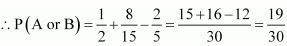Thus, the probability that the selected student has opted for NCC or NSS is  19/30.

(ii) P(not A and not B)
= P(A' and B')
= P(A' ∩ B')
= P(A ∪ B)'  [(A' ∩ B') = (A ∪ B)' (by De Morgan's law)]
= 1 - P(A ∪ B)
= 1 - P(A or B)
= 1 - 19/30
= 11/30
Thus, the probability that the selected students has neither opted for NCC nor NSS is 11/30.

(iii) The given information can be represented by a Venn diagram as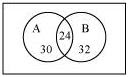It is clear that
Number of students who have opted for NSS but not NCC
n(B – A) = n(B) – n(A ∩ B) = 32 – 24 = 8
Thus, the probability that the selected student has opted for NSS but not for NCC = 8/60 = 2/15